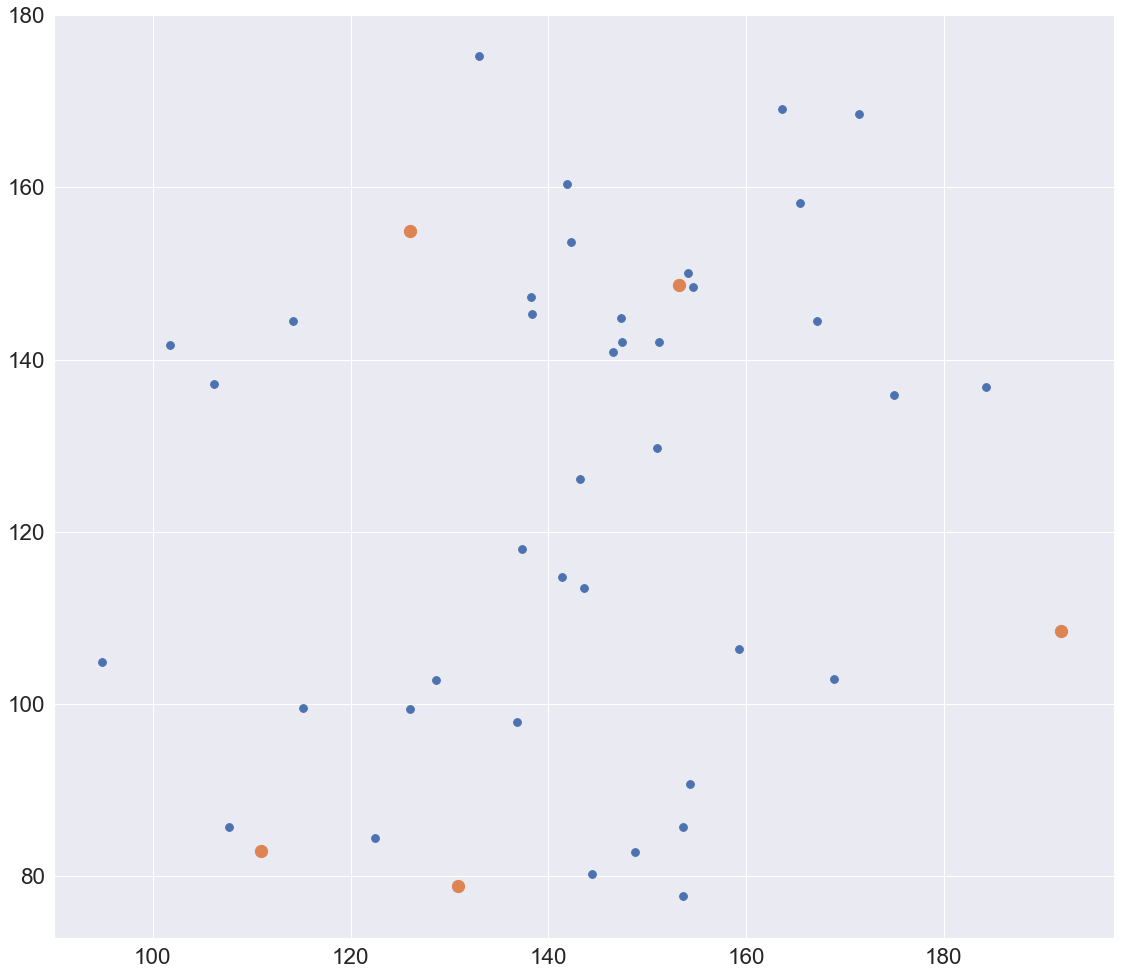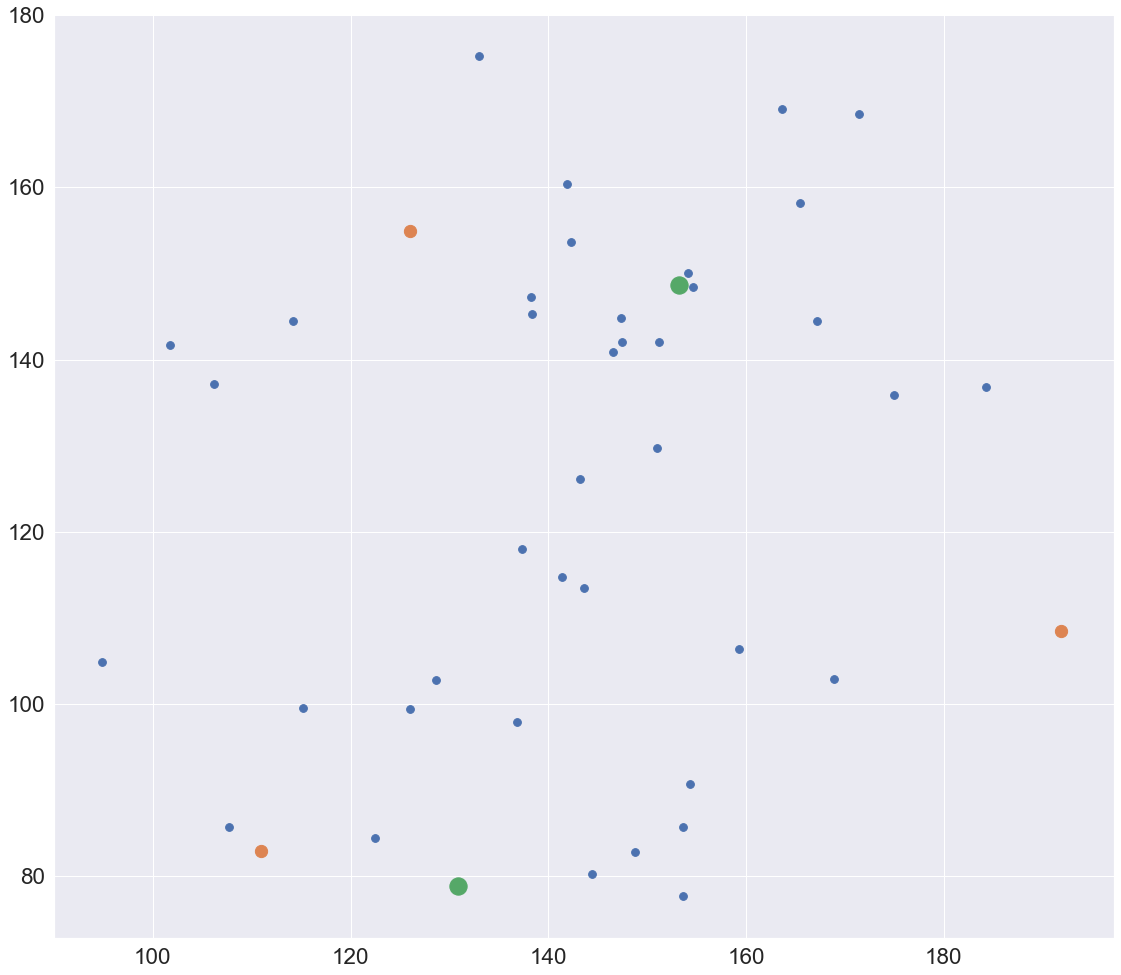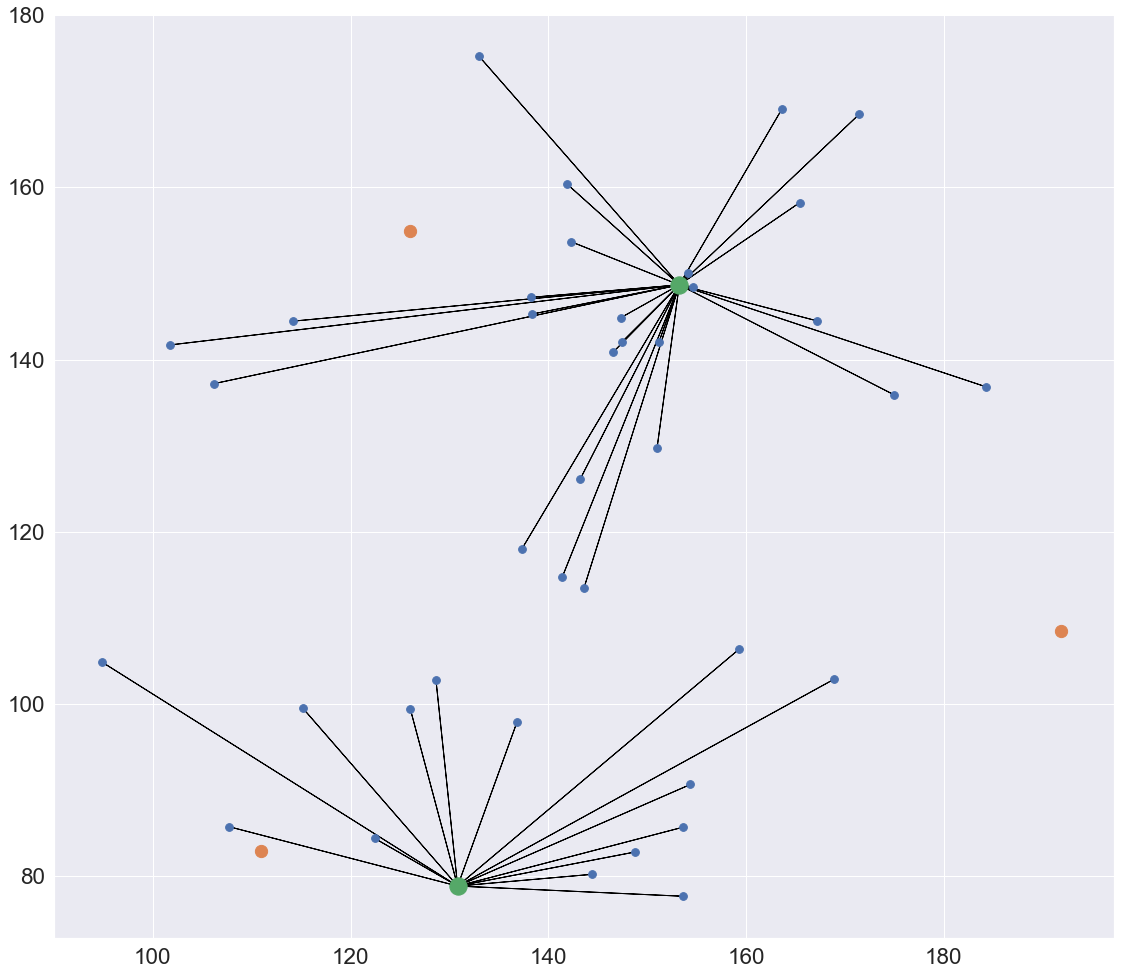# Notebook 5.3 - Multiple Facility Network Location Problem¶

In :
import numpy as np
import matplotlib
import matplotlib.pyplot as plt
import seaborn as sns; sns.set()
from sklearn.datasets import make_blobs

%matplotlib inline

plot_size   = 30
plot_width  = 19
plot_height = 17

params = {'legend.fontsize': 'large',
'figure.figsize': (plot_width,plot_height),
'axes.labelsize': plot_size,
'axes.titlesize': plot_size,
'xtick.labelsize': plot_size*0.75,
'ytick.labelsize': plot_size*0.75,
plt.rcParams.update(params)

In :
num_customer = 40
num_facility = 5

In :
center_box = (100, 200)

cust_coord, _  = make_blobs(n_samples=num_customer,
centers=2,
cluster_std=20,
center_box=center_box,
random_state = 2)

facil_coord, _ = make_blobs(n_samples=num_facility,
centers=1,
cluster_std=30,
center_box=center_box,
random_state = 50)

In :
plt.scatter(cust_coord[:, 0],
cust_coord[:, 1],
s=plot_size*2,
cmap='viridis');

plt.scatter(facil_coord[:, 0],
facil_coord[:, 1],
s=plot_size*5,
cmap='viridis');In :
from pulp import *

prob = LpProblem('prob', LpMinimize)

In :
from scipy.spatial import distance

dist_matrix = distance.cdist(cust_coord, facil_coord, 'euclidean')

In :
S = [('S'+str(i+1)) for i in range(num_facility)]
D = [('C'+str(i+1)) for i in range(num_customer)]

In :
n = 2


We are using the above parameter variable to set the number of facilities to be opened.

In :
d = {customer:{facility:dist_matrix[i][j] for j,facility in enumerate(S)} for i,customer in enumerate(D)}

In :
x = LpVariable.dicts('x', (D,S), lowBound = 0, upBound = 1, cat = LpInteger)
y = LpVariable.dicts('y', S, lowBound = 0, upBound = 1, cat = LpInteger)

In :
prob += lpSum([lpSum([x[i][j]*d[i][j] for j in S]) for i in D])

In :
for i in D:
prob += lpSum([x[i][j] for j in S]) == 1

prob += lpSum([y[j] for j in S]) == n

for i in D:
for j in S:
prob += x[i][j] <= y[j]


Note that in the 2nd constrained we use n to define the number of facilities to be opened.

In :
status = prob.solve()

print(f'STATUS\n{LpStatus[status]}\n')

STATUS
Optimal


In :
chosen_facil = []
for j,facility in enumerate(S):
if y[facility].varValue == 1:
chosen_facil.append(j)
print(f'We will be opening facility {facility}')

chosen_facil

We will be opening facility S2
We will be opening facility S4

Out:
[1, 3]

## Summary¶

from pulp import *
from scipy.spatial import distance

# Calculate distance matrix
dist_matrix = distance.cdist(cust_coord, facil_coord, 'euclidean')

# Initialise problem
prob = LpProblem('prob', LpMinimize)

# Define sets of customers and facilities
n_customers = len(cust_coord)
n_facil     = len(facil_coord)

S = [('S'+str(i+1)) for i in range(num_facility)]
D = [('C'+str(i+1)) for i in range(num_customer)]

# Prepare distance matrix parameters
d = {customer:{facility:dist_matrix[i][j] for j,facility in enumerate(S)} for i,customer in enumerate(D)}

# Define decision variables
x = LpVariable.dicts('x', (D,S), lowBound = 0, upBound = 1, cat = LpInteger)
y = LpVariable.dicts('y', S, lowBound = 0, upBound = 1, cat = LpInteger)

# Objective function
prob += lpSum([lpSum([x[i][j]*d[i][j] for j in S]) for i in D])

# Constraint 1: Each customer will be served by 1 facility
prob += lpSum([y[j] for j in S]) == 1

# Constraint 2: Only 1 facility will be established
prob += lpSum([y[j] for j in S]) == 1

# Constraint 3: Only established facilities can serve customers
for i in D:
for j in S:
prob += x[i][j] <= y[j]

# Solve the model
status = prob.solve()

In :
plt.scatter(cust_coord[:, 0],
cust_coord[:, 1],
s=plot_size*2,
cmap='viridis',
zorder = 10000);

plt.scatter(facil_coord[:, 0],
facil_coord[:, 1],
s=plot_size*5,
cmap='viridis');

plt.scatter(facil_coord[chosen_facil, 0],
facil_coord[chosen_facil, 1],
s=plot_size*10,
cmap='viridis',
zorder=99999)

Out:
<matplotlib.collections.PathCollection at 0x7fb5a856cf90>The above graph plots correctly the facilities to be opened, but how do we know which one serves any customer?

In :
vect_orig = []
vect_dest = []
u = v = np.zeros((len(vect_orig),len(vect_orig)))

for j,facility in enumerate(S):
for i,customer in enumerate(D):
if x[customer][facility].varValue == 1:
vect_orig.append(facil_coord[j])
vect_dest.append(cust_coord[i])

vect_orig = np.array(vect_orig)
vect_dest = np.array(vect_dest)


In the above code we are iterating through our sets of facilities and customers.

Wherever we find a pair that is connected, we will append the origin and destination coordinates of a link between them to vect_orig and vect_dest, respectively.

In :
plt.scatter(cust_coord[:, 0],
cust_coord[:, 1],
s=plot_size*2,
cmap='viridis',
zorder = 10000);

plt.scatter(facil_coord[:, 0],
facil_coord[:, 1],
s=plot_size*5,
cmap='viridis');

plt.scatter(facil_coord[chosen_facil, 0],
facil_coord[chosen_facil, 1],
s=plot_size*10,
cmap='viridis',
zorder=99999)

for i in range(len(vect_orig)):
plt.arrow(vect_orig[i],
vect_orig[i],
vect_dest[i]-vect_orig[i],
vect_dest[i]-vect_orig[i],
color='black')We have now replotted the graph, but with the addition of arrows between facilities and the customers that they serve.

The eagled-eyed among you might have notices that we are now also using the zorder parameter, which allows us to define the order with which the elements of the graph are served.